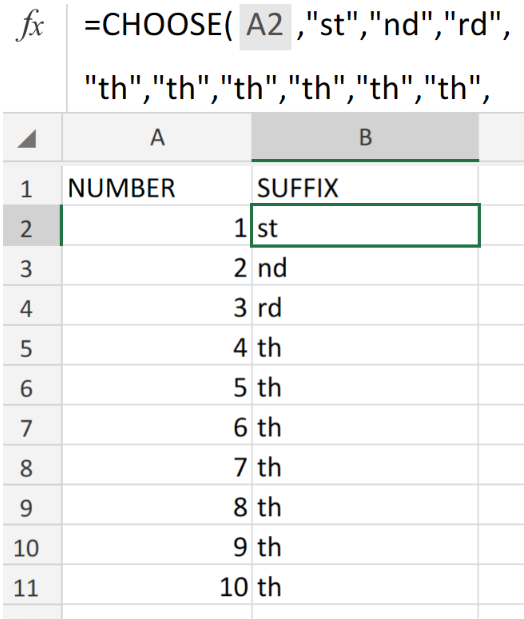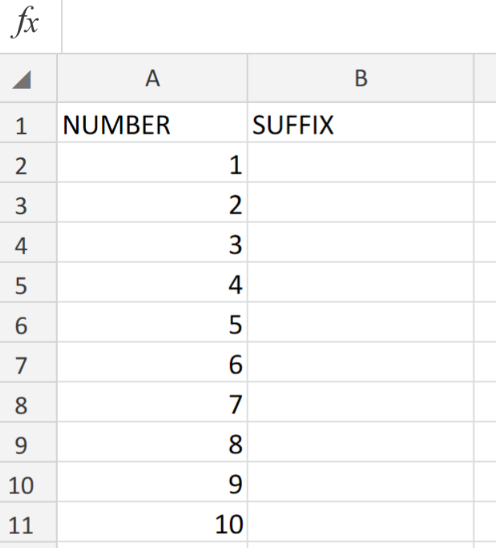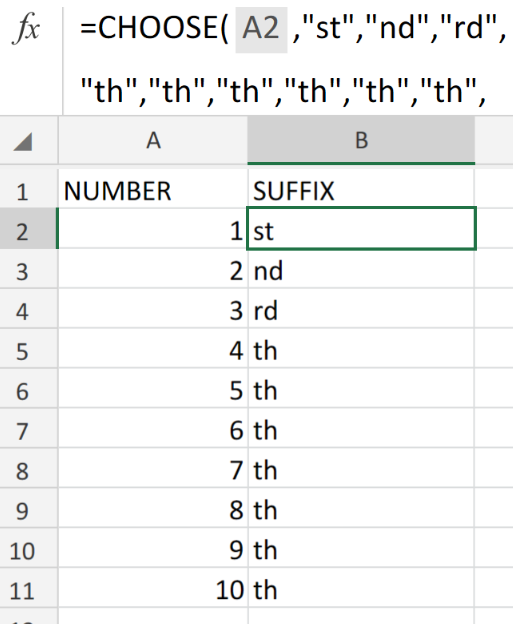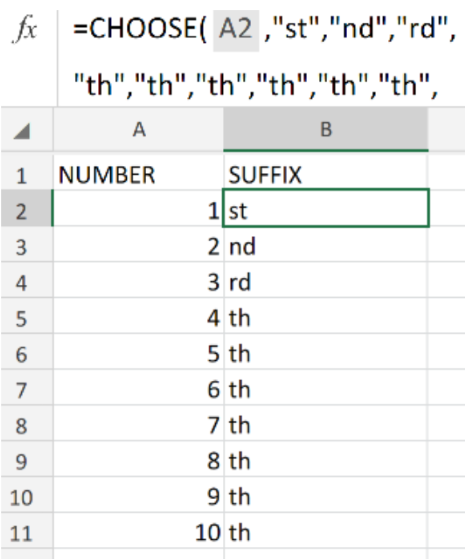Get instant live expert help with Excel or Google Sheets“My Excelchat expert helped me in less than 20 minutes, saving me what would have been 5 hours of work!”

#### Post your problem and you’ll get Expert help in seconds.

Your message must be at least 40 characters
Our professional Expert are available now. Your privacy is guaranteed.

# How Use RANK Combined with the SUFFIX Function in ExcelFigure 1. of Rank with Suffix Function in Excel.

Let’s say we want to add ordered suffixes to a range of numbers in Excel (like, 1st, 2nd, 3rd, etc.), We are going to utilize a formula syntax which is derived from the Excel CHOOSE Function.

The Excel CHOOSE Function will perform the task of assigning the desired suffix to each number value.

## Generic Formula

`=CHOOSE(number,"st","nd","rd","th","th","th","th","th","th","th")`

## How to use the CHOOSE Function in Excel.

The Excel CHOOSE formula syntax operates by returning a letter suffix in specific ranking order for every sequential number value.

We combine each number value with suffix letters by using the Ampersand symbol (&), which is a connection operator symbol in Excel,

We can achieve this by following three simple steps;

1. Arrange the available data in our worksheet by inputting the ordinal NUMBER values in a single column. Label the next column as SUFFIX.

See the example illustrated below;Figure 2. of Ordinal Numbers in Excel.

1. Because Ordinal numbers depict ranking, position or an order of sequence, they are commonly written as a combination of number and a suffix letter:  1st, 2nd, 3rd, etc.

Hence, the formula syntax which we will enter into the formula bar for cell B2 of our worksheet is as follows;

`=CHOOSE(A2,"st","nd","rd","th","th","th","th","th","th","th")`Figure 3. of Rank with Suffix Function in Excel.

1. Copy the formula syntax in cell B2 into every other cell in column B to achieve the desired ranking results.

## Note

The Excel CHOOSE Function is ideal for small set of numbers only, like if the number values are in a Range of 1 up to 10. This is not the solution for larger ranges of numbers.Figure 4. of Final Result.

## Instant Connection to an Expert through our Excelchat Service

Our live Excelchat Service is here for you. We have Excel Experts available 24/7 to answer any Excel questions you may have. Guaranteed connection within 30 seconds and a customized solution for you within 20 minutes

### Did this post not answer your question? Get a solution from connecting with the expert.Another blog reader asked this question today on Excelchat:
Related blogs## Subscribe to Excelchat.coAnother blog reader asked this question today on Excelchat: